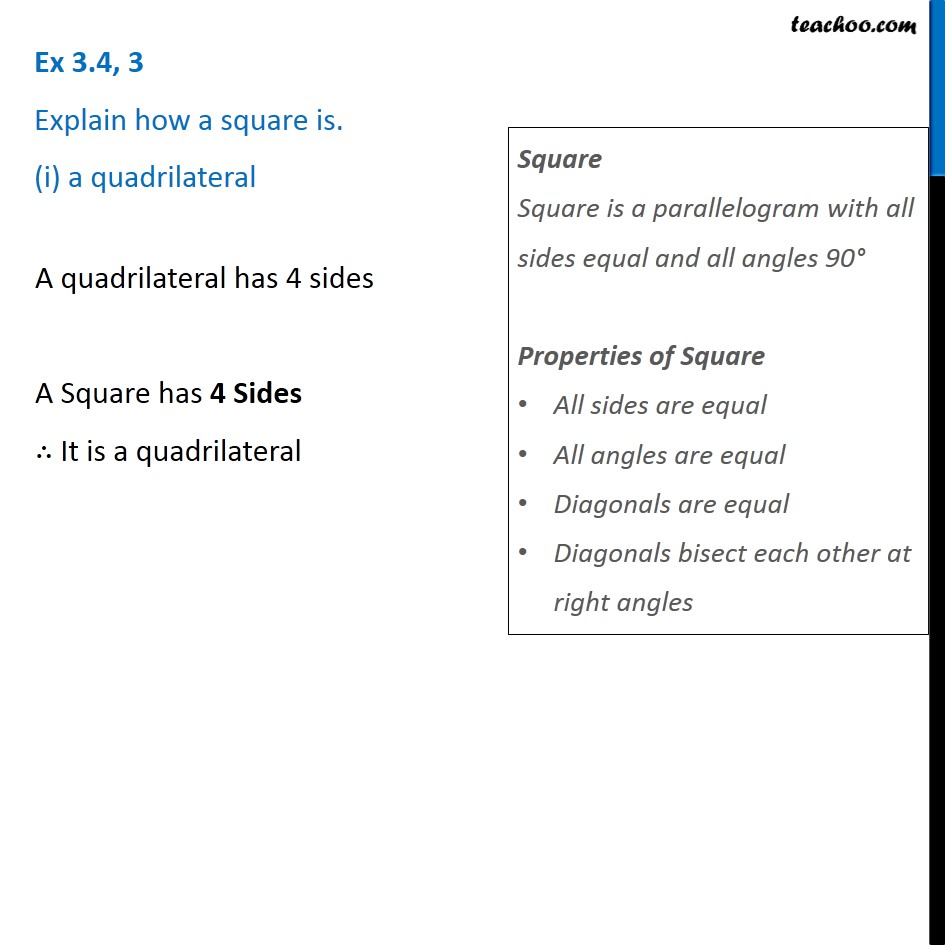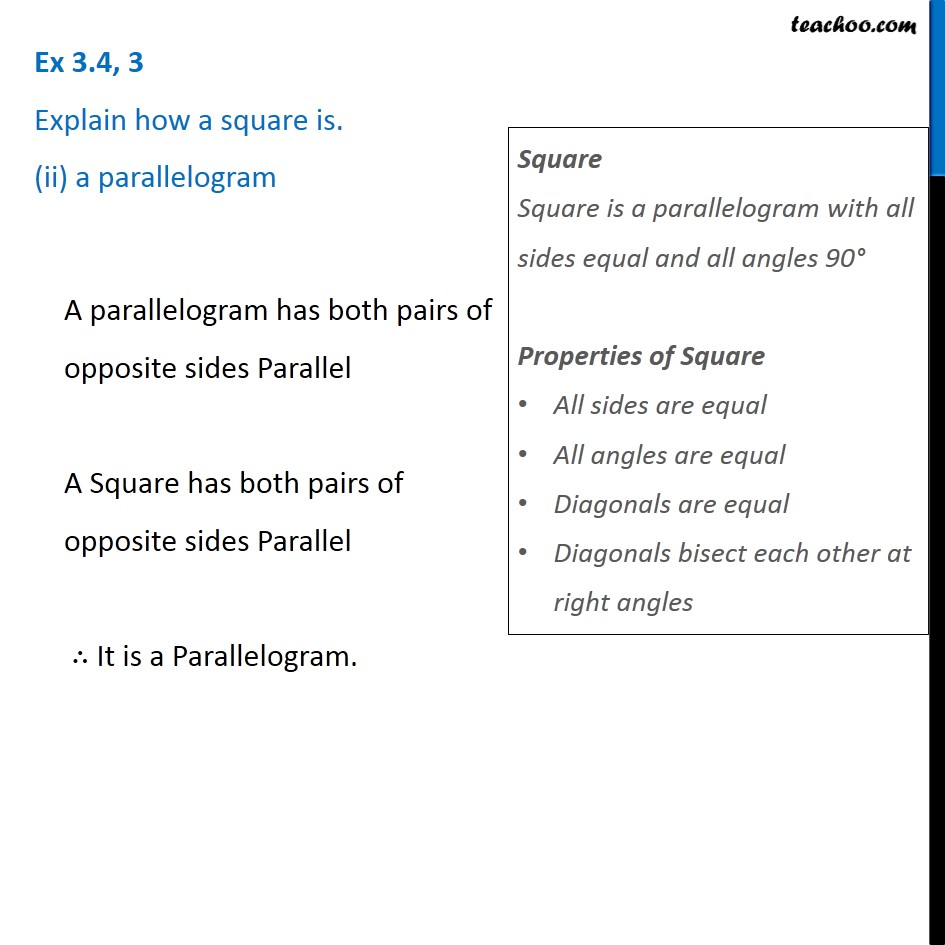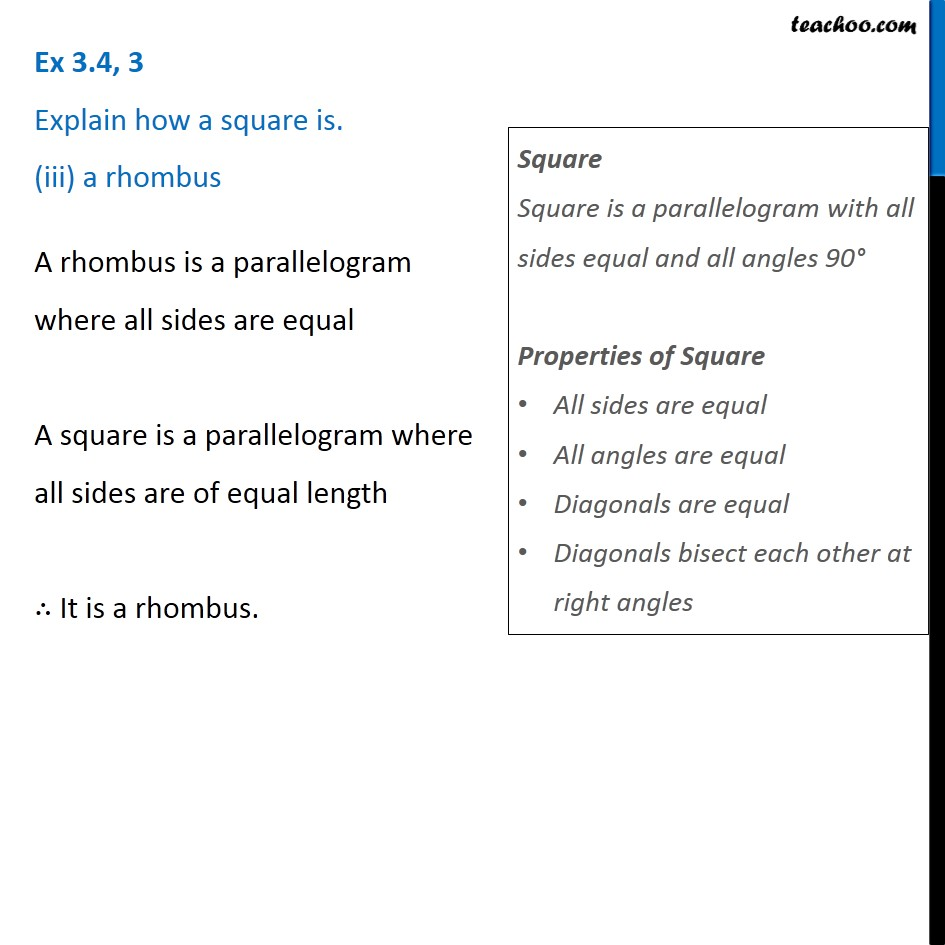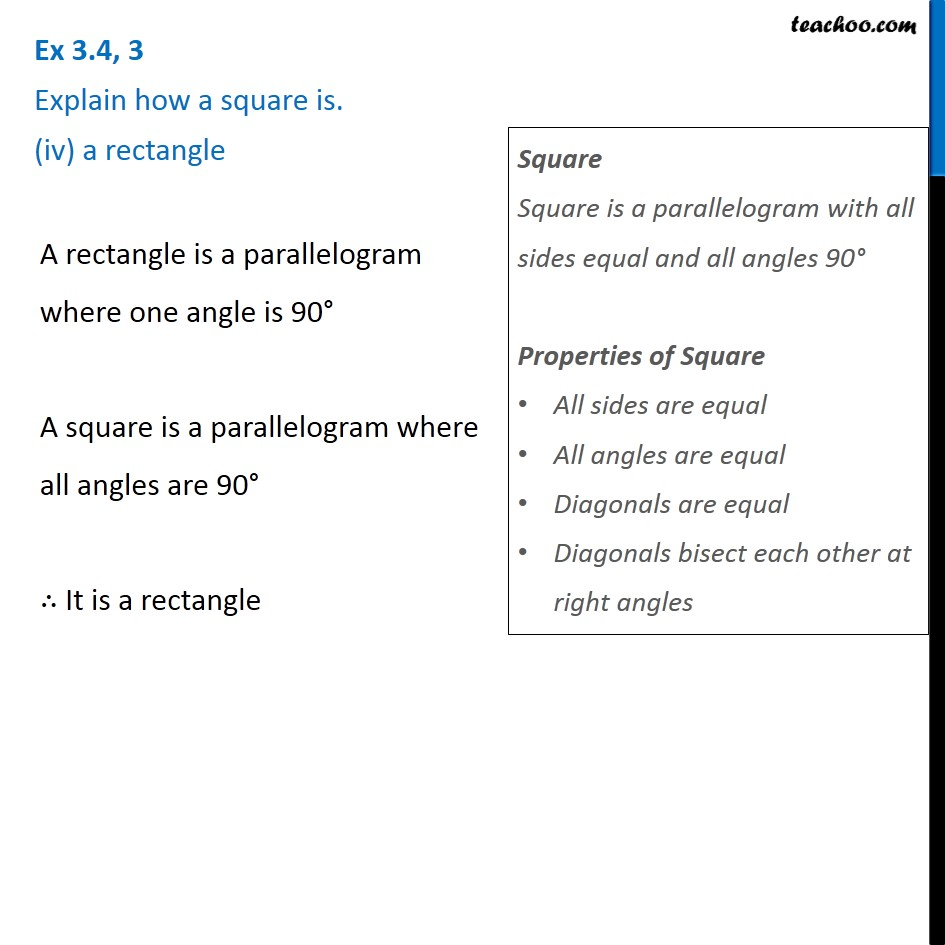Subscribe to our Youtube Channel - https://you.tube/teachoo

1. Chapter 3 Class 8 Understanding Quadrilaterals
2. Concept wise
3. Rhombus, Rectangle, Square

Transcript

Ex 3.4, 3 Explain how a square is. (i) a quadrilateral A quadrilateral has 4 sides A Square has 4 Sides ∴ It is a quadrilateral Square Square is a parallelogram with all sides equal and all angles 90° Properties of Square All sides are equal All angles are equal Diagonals are equal Diagonals bisect each other at right angles Ex 3.4, 3 Explain how a square is. (ii) a parallelogram A parallelogram has both pairs of opposite sides Parallel A Square has both pairs of opposite sides Parallel ∴ It is a Parallelogram. Square Square is a parallelogram with all sides equal and all angles 90° Properties of Square All sides are equal All angles are equal Diagonals are equal Diagonals bisect each other at right angles Ex 3.4, 3 Explain how a square is. (iii) a rhombus A rhombus is a parallelogram where all sides are equal A square is a parallelogram where all sides are of equal length ∴ It is a rhombus. Square Square is a parallelogram with all sides equal and all angles 90° Properties of Square All sides are equal All angles are equal Diagonals are equal Diagonals bisect each other at right angles Ex 3.4, 3 Explain how a square is. (iv) a rectangle A rectangle is a parallelogram where one angle is 90° A square is a parallelogram where all angles are 90° ∴ It is a rectangle Square Square is a parallelogram with all sides equal and all angles 90° Properties of Square All sides are equal All angles are equal Diagonals are equal Diagonals bisect each other at right angles

Rhombus, Rectangle, Square